# 【Python基础】第十课：DataFrame的相关操作

## 数据选取，新增数据，删除数据，数据的索引

Posted by x-jeff on February 2, 2020

# 1.前言

1
2
3
import pandas as pd
df=pd.DataFrame([['Frank','M',29],['Mary','F',23],['Tom','M',35],['Ted','M',33],['Jean','F',21],['Lisa','F',20]])
df.columns=['Name','Gender','Age']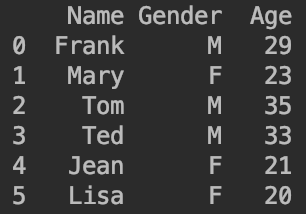# 2.数据的选取

【Python基础】第六课：处理CSV、Excel格式的数据中读取csv、excel的数据一样，我们可以使用.loc.iloc来选取数据。

## 2.1.选择特定条件下的数据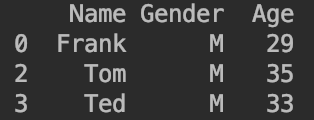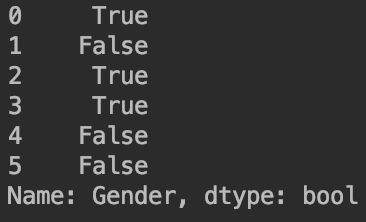• 女性并且年龄大于等于21的数据：df[(df['Gender']=='F') & (df['Age']>=21)]
• 女性或者年龄大于22的数据：df[(df['Gender']=='F') | (df['Age']>22)]

# 3.数据的新增和删除

## 3.1.新增数据

### 3.1.1.新增列

df['Employee']=True：新增Employee列，该列的值全部为True。

df['Level']=[1,2,3,4,5,6]：新增Level列，该列的值依次为1,2,3,4,5,6。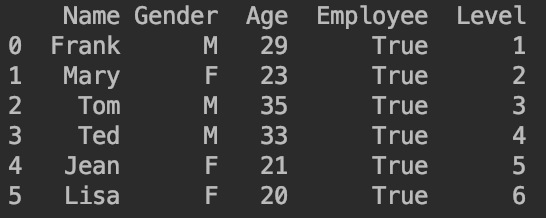### 3.1.2.新增行

df.loc={'Name':'Wade','Gender':'M','Age':28,'Employee':True,'Level':7}：原DataFrame的列标签必须都被赋值，但是顺序不必一致。

⚠️这里不能用df.iloc={'Name':'Wade','Gender':'M','Age':28,'Employee':True,'Level':7}

1
df = df.append(pd.DataFrame([{'Name':'James','Gender':'M','Age':32,'Employee':True,'Level':8}]),ignore_index=True,sort=False)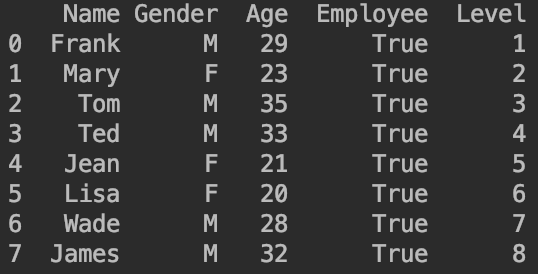⚠️sort=False指的是列标签不用重新排序（按照首字母排序）。ignore_index=True指的是新增的行的索引号不重新排序。

1
df = df.append(pd.DataFrame([{'Name':'James','Gender':'M','Age':32,'Employee':True,'Level':8}]),ignore_index=False,sort=True)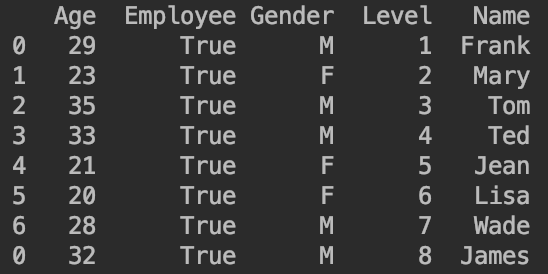## 3.2.删除数据

### 3.2.2.方法二：.drop

⚠️axis=1对列操作；axis=0对行操作。这个设定和Numpy中刚好相反。

# 4.数据的索引

1
2
df['UserID']=range(101,108)
df.set_index('UserID',inplace=True)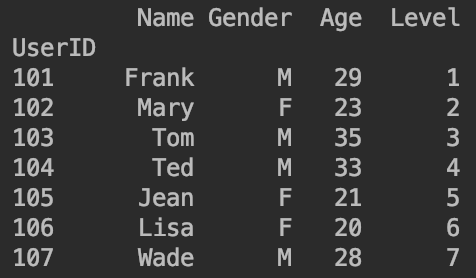❗️可以用df.loc或者df.iloc进行取值。

range(a,b)可以生成：a,a+1,a+2,…,b-1。其中，a,b必须为整数
range(a,b,step)step指的是步长。例如range(1,11,2)生成的数为1,3,5,7,9。

# 5.代码地址